Latest Banking jobs   »

# Reasoning Ability Quiz For IDBI AM/ Bank of India PO 2023 – 04th April

Directions (1-5): In each of the question, relationships between some elements are shown in the statements(s). These statements are followed by conclusions numbered I and II. Read the statements and give the answer.
(a) If only conclusion I follows.
(b) If only conclusion II follows.
(c) If either conclusion I or II follows.
(d) If neither conclusion I nor II follows.
(e) If both conclusions I and II follow.

Q1. Statements: C < M >N = T, C ≤ V, M > R
Conclusions: I. N > R II. V > T

Q2. Statements: D > F = G > H = I ≤ J
Conclusions: I. F > I II. J ≥ H

Q3. Statements: Z < K < O > P, K< M
Conclusions: I. M > P II. O >Z

Q4. Statements: C ≥ D< T = F ≥ G, C < W
Conclusions: I. T =G II. G< T

Q5. Statements: R < T < S < P > Q, R> X
Conclusions: I. S < Q II. X < S

Directions (6-8): Study the information carefully and answer the questions given below.
Point P is 15m west of point Q. Point R is 5m east of point S. Point U is 10m north of point V. Point Q is 10m north of point R. Point U is 10m east of point T, which is 5m north of S.

Q6. What is the shortest distance between point P and Point V?
(a) 24m
(b) 25m
(c) √624m
(d) 20m
(e) None of these

Q7. In which direction point U with respect to point P?
(a) North-east
(b) North
(c) South-east
(d)South-west
(e) None of these

Q8. In which direction point Q with respect to point V?
(a) North
(b) North-east
(c) North-west
(d)South-east
(e) None of these

Q9. Meena faces west and walks 2 km to reach P, then turn left walks 5km. After this, she turns right and walks 5 km again. Now, she turns again towards right and walks 4km, then turn right and walks 5km. Now what is the shortest distance between Point P and Final position and in which direction she is with respect to P?
(a) 1km, North
(b) 2km, North east
(c) 1km, South
(d) 2km, south west
(e) None of these

Rohit starts walking from point U, he walks 10m in the west direction and reached at V, after that he turns his right and walks 15m to reach at point Z. From point Z, he turns to his right and walks 8m to reach at point F. Now, he starts walks 18m in south direction and reached at point X.

Q10. If point M is 2m west of point U, then what is the shortest distance between point Z and M?
(a) 17m
(b) 19m
(c) 16m
(d) 18m
(e) None-of-these

Q11. In which direction point F with respect to point U?
(a) North-west
(b) North
(c) South
(d) West
(e) North east

Direction (12-13): Read the information carefully and answer the questions given below:
Point B is south of point C which is 5m west of point G. Point E is 3m south of point B. Point C is 13m north-east of point A. Point F is 5m east of point E. Point A is 12m west of point B.

Q12. Point C is in which direction with respect to point F?
(a) North
(b) South-West
(c) North-east
(d) North-west
(e) None of these

Q13. What is the shortest distance between point C and B?
(a) 4m
(b) 6m
(c) 5m
(d) 10m
(e) None of these

Directions (14-15): Study the information given below carefully and answer the questions that follow:
Point C is 2 m north of Point B. Point A is 1 m east of B and Point H is 2 m south of A. Point G is 1 m west of point H while Point D is 3 m east of point G and Point F is 2 m north of point D. Point E is just in middle of point H and point D.

Q14. If Point K is 2m north of point F, then what is the shortest distance between point G to point K?
(a) 8m
(b) 7m
(c) 10m
(d) 5m
(e) None of these

Q15. In which direction is point C with respect to D?
(a) North-East
(b) North-West
(c) South-East
(d) West
(e) None of these

Solutions

Solutions (1-5):
S1. Ans.(d)
Sol. I. N > R (False) II. V > T (False)
S2. Ans.(e)
Sol. I. F > I (True) II. J ≥ H (True)
S3. Ans.(b)
Sol. I. M > P (False) II. O >Z (True)
S4. Ans.(c)
Sol. I. T =G (False) II. G < T (False)
S5. Ans.(b)
Sol. I. S < Q (False) II. X < S (True)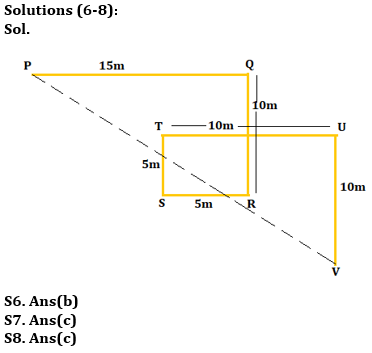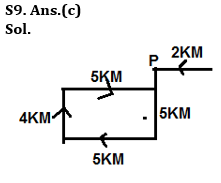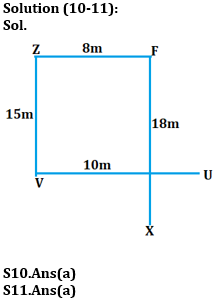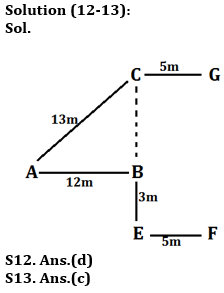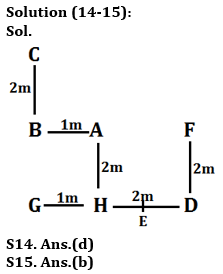## FAQs

### What is the selection process of the IDBI AM?

The selection process of IDBI AM is Online exam and Interview.

#### Congratulations!Union Budget 2023-24: Free PDF